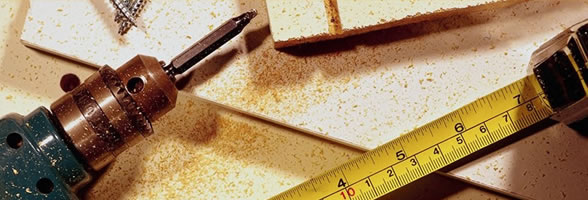## KNOWLEDGE HUB

11/08/2013 16:04

### DO YOU KNOW THAT ???????

The method of getting the square of a number which has the last digit 5 is as follows: Add 1 to the number which is before 5 and multiply it with the same number and add 25. Example: (75)^2= 7*8=56. so the required no. is 5625.

—————

—————

## SYLLABUS

28/08/2013 19:14

### MATHS HONS

fyup.du.ac.in/disciplinecourses/Maths/Mathematics_4_yr.pdf#DCI

—————

19/08/2013 17:32

### BMA

I. Numbers (6 lectures) Prime numbers Interesting properties of prime numbers without proofs Ramanujan's work on the Prime Number Theorem Euclid's division algorithm Mathematical illustration through intuitive examples Visualisation through tiling analogy Encryption and Prime...

—————

04/09/2013 11:24